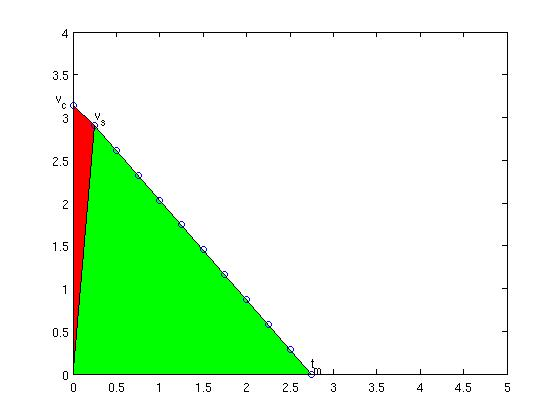# EMC2 tpRunCycle revisited

I started this EMC2 wiki page in 2006 when trying to understand how trajectory control is done in EMC2. Improving the trajectory controller is a topic that comes up on the EMC2 discussion list every now and then. The problem is just that almost nobody actually takes the time and effort to understand how the trajectory planner works and documents it...

A recent post on the dev-list has asked why the math I wrote down in 2006 isn't what's in the code, so here we go:I will use the same shorthand symbols as used on the wiki page. We are at coordinate P ("progress") we want to get to T ("target") we are currently travelling at vc ("current velocity"), the next velocity suggestion we want to calculate is vs ("suggested velocity), maximum allowed acceleration is am ("max accel") and the move takes tm ("move time") to complete. The cycle-time is ts ("sampling time"). The new addition compared to my 2006 notes is that now the current velocity vc as well as the cycle time ts is taken into account.

As before, the area under the velocity curve is the distance we will travel, and that needs to be equal to the distance we have left, i.e. (T-P). (now trying new latex-plugin for math:) (EQ1)

Note how the first term is the area of the red triangle and the second therm is the area of the green triangle. Now we want to calculate a new suggested velocity vs so that using the maximum deceleration our move will come to a halt at time tm, so(EQ2):

Inserting this into the first equation gives (EQ3):

with the solution (we obviously want the plus sign)(EQ5)

which we can insert back into EQ2 to get (EQ6) (this is the new suggested max velocity. we obviously apply the accel and velocity clamps to this as noted before)

It is left as an (easy) exercise for the reader to show that this is equivalent to the code below (note how those silly programmers save memory by first using the variable discr for a distance with units of length and then on the next line using it for something else which has units of time squared):

discr = 0.5 * tc-&gt;cycle_time * tc-&gt;currentvel - (tc-&gt;target - tc-&gt;progress); discr = 0.25 * pmSq(tc-&gt;cycle_time) - 2.0 / tc-&gt;maxaccel * discr; newvel = maxnewvel = -0.5 * tc-&gt;maxaccel * tc-&gt;cycle_time +tc-&gt;maxaccel * pmSqrt(discr);

over and out.

## 4 thoughts on “EMC2 tpRunCycle revisited”

1.Rick says:

Can i Ask some questions about EMC2 TP? I have an A X Y (A :rotary about x) machine but cant manage to get a proper blending, if you have some spare time i will apreciate,
regards
rick

2.admin says:

Hi Rick,
I haven't looked much at blending, nor described it here or in the linuxcnc documentation. So I don't know that much about it.
You will get a much wider audience for your question on the linuxcnc mailing-list(s) at:
https://lists.sourceforge.net/lists/listinfo/emc-users
I'm sure everyone is interested if you have uncovered a bug!

Anders

3.Rick says:

many thanks, good luck at cycling
regards
rick

This site uses Akismet to reduce spam. Learn how your comment data is processed.